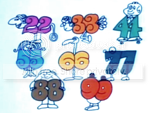1

## 11/11/11

Published on Thursday, November 10, 2011 in , , ,This Friday is 11-11-11. It is, of course, Rememberance Day. Many others, are simply taking advantage of the once-in-a-lifetime date as a reason to celebrate, including countless couples who are getting married on that date.

As I did for 9/9/9 here and here, and for 10/10/10 here, I'll be marking the day by looking at the qualities of the number 11, and what kind of fun we can have with it.

Many people's first introduction to the idea that math can be done in your head comes when they learn about multiplying single-digit numbers by 11, as seen in this classic Schoolhouse Rock video:

When you're first learning multiples of 11, you do quickly notice that the nice neat double-the-digit pattern quickly ends after 9. The ease of learning to multiply by 11 in your head doesn't have to end there, however! There's another pattern that, once learned, makes it easy to multiply numbers of ANY size! The best place to learn this technique is via Math World's Math Tricks: Multiply by 11 post, as it includes not only the teaching video below, but some practice exercises, as well.

Demonstrating this ability can be very impressive to an audience, especially since many of them will remember struggling with multiples of 11 beyond 9. One of the best presentations I've seen for demonstrating your ability to quickly multiply by 11 is "The Mental Block" from Paul Brook's book, Chrysalis of a Polymath. With that presentation, the audience member remembers that they brought up 11 (no force, but some very effective psychology), and they time you as you quickly multiply six different two-digit numbers by 11. The whole performance is presented while discussing how people's aversion to math is often a bigger barrier than lack of ability.

Surprisingly, there's also a simple trick for dividing any two-digit number by 11, and giving the answer to three decimal places. It's even simpler, but it's less well-known, and more impressive, due to the seeming mental calculation of multiple decimals.

1/11 is a repeating decimal equal to .090909... and so on. This repetition of 9 makes determining the decimal equivalent of any “11th” easy:

1/11=.090909...
2/11=.181818...
3/11=.272727...
4/11=.363636...
5/11=.454545...
6/11=.545454...
7/11=.636363...
8/11=.727272...
9/11=.818181...
10/11=.909090...

If you know your multiples of 9 up to 10, this is a simple matter. If not, you can get some quick help from my Magic of 9 post.

Knowing this, dividing any two-digit number by 11 in your head becomes a simple matter. Let's divide 63 by 11. First, what's the largest multiple of 11 equal to or lower than the chosen number? In our example, this would be 55. This tells you that the answer will by 5 and some decimal. Next, subtract that multiple of 11 from the given number. We subtract 63 - 55 = 8, so you could give the answer as 5 8/11, but there's a more impressive way to give this answer.

Knowing the pattern of 11th above relies on 9, ask yourself what is 8 * 9? It's 72, so you know the decimal for 8/11 equals .727272..., so you could give the answer for 63/11 as 5.727272... giving the endless decimal pattern. Even better, I suggest limiting the answer to three decimal places, giving the answer in this case as 5.727. Sure, the person verifying your calculation will still see the .727272... pattern, but it will be less apparent and more impressive to the rest of your audience!

If you'd like to be able to divide any two-digit number by any number from 1-11, check out my Mental Division With Decimal Precision post. Alternatively, Blake over at Nerd Paradise turns this feat around, and allows you to give a fraction for any given two- or three-digit repeating decimal.

Going back to the multiplication of 11, there's an even better way to demonstrate your ability to multiply by 11 while hiding that you have to do this with 11. As taught in episode 170 of Scam School, your multiplication ability is hidden behind an addition problem:

The larger the numbers you can multiply by 11, the greater the freedom you'll be able to give to people in choosing their initial numbers. If you can multiply numbers up to and including 1,287 by 11, you can let the other person choose any two numbers from 1-99 as the starting numbers. You can learn more about the working behind this trick in my Scam School Mathemagic post.

We'll wrap this up with James Grime's 11-11-11 video, the first full official video done for the new NumberPhile YouTube channel, talking about all things 11, including an amazing fact about books:

Sadly, I'll only get once more chance to celebrate a day like this in my lifetime, which is next year on 12/12/12.

### 1 Response to 11/11/11Anonymous

Sadly, I'll only get once more chance to celebrate a day like this in my lifetime, which is next year on 12/12/12.

Well since the mayans say the world will end 9 days after that anyway, I do not thiink it makes much difference.

/Seriously though fun article, nice job.